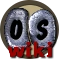Template documentation [purge]
This documentation is transcluded from Template:RuneReq/doc.

The RuneReq template displays a list of runes and numbers against them. Used for spells that give a list of number of runes needed.

## Usage

```{{RuneReq
| Air      = number of Air runes
| Water    = number of Water runes
| Earth    = number of Earth runes
| Fire     = number of Fire runes
| Mind     = number of Mind runes
| Body     = number of Body runes
| Astral   = number of Astral runes
| Chaos    = number of Chaos runes
| Cosmic   = number of Cosmic runes
| Nature   = number of Nature runes
| Death    = number of Death runes
| Law      = number of Law runes
| Blood    = number of Blood runes
| Soul     = number of Soul runes
| Wrath    = number of Wrath runes
| Orb      = number of Uncharged Orbs
| Banana   = number of Bananas
}}
```

## Example

The following example:

`{{RuneReq|Astral=2|Earth=1}}`